TitleCollege Algebra
Tutorial 11: Complex Rational ExpressionsLearning Objectives

 After completing this tutorial, you should be able to: Simplify complex fractions.Introduction

 Are fractions getting you down?  If so, you are not alone.  Well, you are in luck. We have one more tutorial devoted to rational expressions (fractions).  In this tutorial we will be looking at simplifying complex fractions.  If you need a review on simplifying and dividing rational expressions, feel free to go back to Tutorial 9: Multiplying and Dividing Rational Expressions. If you need a review on finding the LCD of fractions feel free to go back to Tutorial 10: Adding and Subtracting Rational Expressions. It is time to get started with this tutorial.Tutorial

 Complex Fraction

 A complex fraction is a rational expression that has a fraction in its numerator, denominator or both. In other words, there is at least one small fraction within the overall fraction. Some examples of complex fractions are: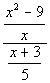andThere are two ways that you can simplify complex fractions.  We will call them method I and method II.

 Method I  Simplifying a Complex Fraction

 In other words, you will be combining all the parts of the numerator to form one fraction and all of the parts of the denominator to form another fraction.  If you need a review on adding and subtracting rational expressions, go to Tutorial 10: Adding and Subtracting Rational Expressions.

 A fraction bar is the same as a division bar where you are taking the numerator divided by the denominator.  Recall that when you divide by a fraction it is the same as multiplying by its reciprocal.  So in this step you will be multiplying the numerator by the reciprocal of the denominator. If you need a review on dividing rational expressions, go to Tutorial 9: Multiplying and Dividing Rational Expressions.

Step 3: If needed, simplify the rational expression.

 If you need a review on simplifying rational expressions, go to Tutorial 8: Simplifying Rational Expressions.Example 1: Simplify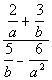.

 Step 1:   If needed, rewrite the numerator and denominator so that they are each a single fraction.

 Combining only the numerator we get: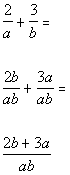*Rewrite fractions with LCD of ab

 Combining only the denominator we get: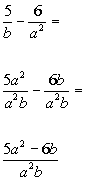*Rewrite fractions with LCD of a^2 b

 Putting these back into the complex fraction we get: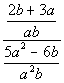*Write numerator over denominator

 AND Step 3: If needed, simplify the rational expression.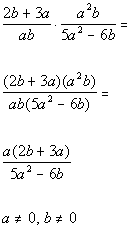*Rewrite div. as mult. of reciprocal     *Divide out a common factor of ab         *Excluded values of the original den.

 Note that the value that would be excluded from the domain is 0.  This is the value that makes the original denominator equal to 0.Example 2: Simplify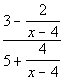.

 Step 1:   If needed, rewrite the numerator and denominator so that they are each a single fraction.

 Combining only the numerator we get: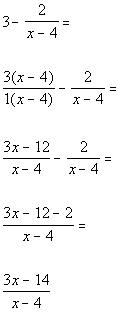*Rewrite fractions with LCD of (x - 4)

 Combining only the denominator we get: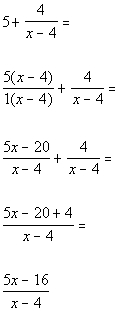*Rewrite fractions with LCD of (x - 4)

 Putting these back into the complex fraction we get: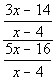*Write numerator over denominator

 AND Step 3: If needed, simplify the rational expression.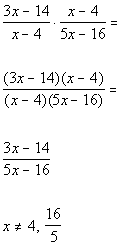*Rewrite div. as mult. of reciprocal       *Divide out a common factor of (x - 4)       *Excluded values of the original den.

 Note that the values that would be excluded from the domain are 4 and 16/5.  These are the values that make the original denominators equal to 0.

 Method II  Simplifying a Complex Fraction

Step 1: Multiply the numerator and denominator of the overall complex fractions by the LCD of the smaller fractions.

 Recall that when you multiply the exact same thing to the numerator and the denominator, the result is an equivalent fraction.  If you need a review on finding the LCD, go back to Tutorial 10: Adding and Subtracting Rational Expressions.

Step 2: If needed, simplify the rational expression.Example 3: Simplify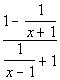.

 Step 1: Multiply the numerator and denominator of the overall complex fractions by the LCD of the smaller fractions.

 The denominator of the numerator's fraction has the following factor: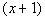The denominator of the denominator's fraction  has the following factor: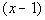Putting all the different factors together and using the highest exponent, we get the following LCD for all the small fractions: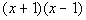Multiplying numerator and denominator by the LCD we get: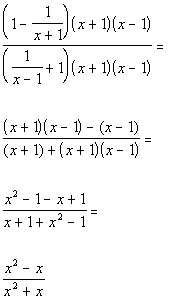*Mult. num. and den. by (x + 1)(x - 1)

 Step 2: If needed, simplify the rational expression.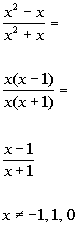*Divide out the common factor of x       *Excluded values of the original den.

 Note that the values that would be excluded from the domain are -1, 1, and 0.  These are the values that make the original denominator equal to 0.Example 4: Simplify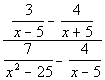.

 Step 1: Multiply the numerator and denominator of the overall complex fractions by the LCD of the smaller fractions.

 The denominators of the numerator's fractions have the following factors: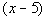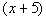The denominators of the denominator's fractions  have the following factors: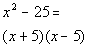Putting all the different factors together and using the highest exponent, we get the following LCD for all the small fractions: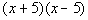Multiplying numerator and denominator by the LCD we get: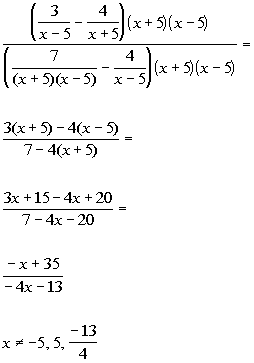*Mult. num. and den. by (x + 5)(x - 5)                               *Excluded values of the original den.

 Note that the values that would be excluded from the domain are -5, 5, and -13/4.  These are the values that make the original denominators equal to 0.

 Step 2: If needed, simplify the rational expression.

 This rational expression cannot be simplified down any farther.Practice Problems

 These are practice problems to help bring you to the next level.  It will allow you to check and see if you have an understanding of these types of problems. Math works just like anything else, if you want to get good at it, then you need to practice it.  Even the best athletes and musicians had help along the way and lots of practice, practice, practice, to get good at their sport or instrument.  In fact there is no such thing as too much practice. To get the most out of these, you should work the problem out on your own and then check your answer by clicking on the link for the answer/discussion for that  problem.  At the link you will find the answer as well as any steps that went into finding that answer.Practice Problems 1a - 1b: Simplify.

 1a.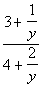(answer/discussion to 1a) 1b.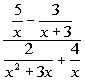(answer/discussion to 1b)Need Extra Help on these Topics?

The following are webpages that can assist you in the topics that were covered on this page:

 http://www.wtamu.edu/academic/anns/mps/math/mathlab/int_algebra/ int_alg_tut34_complex.htm This webpage helps you with simplifying complex rational expressions. http://www.purplemath.com/modules/compfrac.htm This webpage steps through simplifying complex fractions.

Go to Get Help Outside the Classroom found in Tutorial 1: How to Succeed in a Math Class for some more suggestions.

Videos at this site were created and produced by Kim Seward and Virginia Williams Trice.
Last revised on Dec. 15, 2009 by Kim Seward.